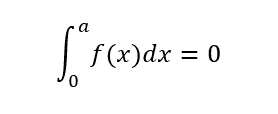# I need some help with integrals

• Kolika28

## Homework Statement

You are given the function

f(x)=3x^2-4x-8

a) Find the values of a.Explain the answers using the function.

## The Attempt at a Solution

a^3-2*a^2-8*a=0
a=-2 v a=0 v a=4

I found the answers, but I don't know how to explain my answers by using the function. What does the zeros of the orginal function has to do with the function I integrated?
Thank you so much for help

Last edited:

## Homework Statement

You are given the function

f(x)=3x^2-4x-8

a) Find the values of a.
View attachment 230840
Explain the answers using the function.

## The Attempt at a Solution

a^3+2*a^2+8*a=0
a=-2 v a=0 v a=4[/B]

I found the answers, but I don't know how to explain my answers by using the function. What does the zeros of the orginal function has to do with the function I integrated?
Thank you so much for help

What's the most common interpretation of an integral?

Hmm, it gives you the area under the curve of a function?

Hmm, it gives you the area under the curve of a function?

It does indeed!

I'm sorry, but I still don't see the importance of the zeros . I'm a slow learner

I'm sorry, but I still don't see the importance of the zeros . I'm a slow learner

Draw a graph of the function ##f##. Alternatively, what might a total area of ##0## mean?

View attachment 230841
I have drawn the function. An area of zero means that it has to be two areas, one over x-axis, and one under, with the same area?

Yes, exactly. Starting from the y-axis in this case. If you changed the lower limit on the integral from ##0##, then you would be calculating the area from a different starting point.

## Homework Statement

You are given the function

f(x)=3x^2-4x-8

a) Find the values of a.
View attachment 230840
Explain the answers using the function.

## The Attempt at a Solution

a^3+2*a^2+8*a=0
a=-2 v a=0 v a=4[/B]

I found the answers, but I don't know how to explain my answers by using the function. What does the zeros of the orginal function has to do with the function I integrated?
Thank you so much for help

If you wrote ##f(x)## correctly, then your integral ##F(a) =a^3 + 2 a^2 + 8a## is incorrect. Your written ##F(a)## has roots ##a = 0, -1 \pm i \sqrt{7}.##

•Kolika28
Ohh, that gives so much sense. Thank you so much, PeroK. I have one last question, it's not a part of the task, but I'm just wondering. If I were to mark both of areas, how would I do it by looking at the function?

I'm sorry, Ray Vickson. I made a mistake when writing the equation here on the chat, the answer are correct though. I will fix it now.

Ohh, that gives so much sense. Thank you so much, PeroK. I have one last question, it's not a part of the task, but I'm just wondering. If I were to mark both of areas, how would I do it by looking at the function?

I'm not sure that you mean. You can see with this function that it's not actually very easy to compare the different areas. In the end, computing the integral is the best way to compare areas, for all but the simplest functions.

I mean, if I were to express both of the areas with an integral, what would the limits on the integrals be? Would one of them have limits -2 and 0, and the other one would have the limits 0 and 4. I know that's wrong, but how am I supposed to find the limits?

I mean, if I were to express both of the areas with an integral, what would the limits on the integrals be? Would one of them have limits -2 and 0, and the other one would have the limits 0 and 4. I know that's wrong, but how am I supposed to find the limits?

What you found was that the integral from ##-2## to ##0## is zero; and, the integral from ##0## to ##4## is zero.

You can more or less see this from the graph.

What you found was that the integral from ##-2## to ##0## is zero; and, the integral from ##0## to ##4## is zero.

You can more or less see this from the graph.
I understand now. Thank you very much for your help, and not least for your patience. It takes some time for me to understand math, but you really helped me.